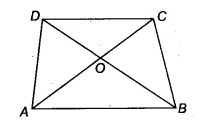Q

# Diagonals AC and BD of a trapezium ABCD with AB || DC intersect each other at O. Prove that ar (AOD) = ar (BOC).

Q : 10    Diagonals AC and BD of a trapezium ABCD with    intersect each other at O. Prove that  .

ViewsWe have a trapezium ABCD such that AB || CD and it's diagonals AC and BD intersect each other at O
ABD and  ABC are on the same base AB and between same parallels AB and CD
ar(ABD) = ar (ABC)

Now, subtracting AOB from both sides we get

ar ( AOD) = ar ( BOC)

Hence proved.

Exams
Articles
Questions